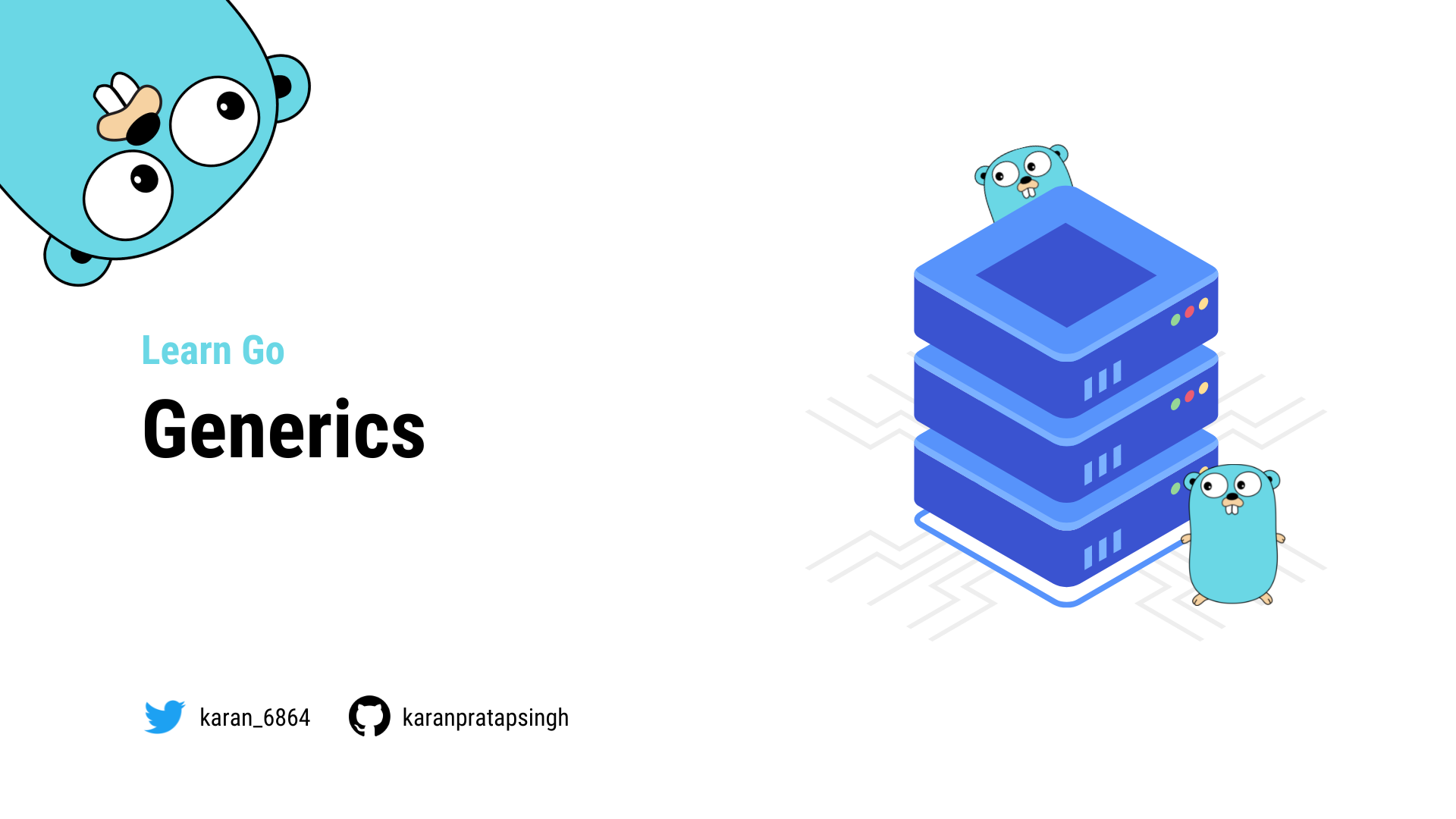# Generics

Authors
•Name
Karan Pratap Singh
@karan_6864In this section, we will learn about Generics which is a much awaited feature that was released with Go version 1.18.

## What are Generics?

Generics means parameterized types. Put simply, generics allow programmers to write code where the type can be specified later because the type isn't immediately relevant.

Let's take a look at an example to understand this better.

For our example, we have simple sum functions for different types such as `int`, `float64`, and `string`. Since method overriding is not allowed in Go we usually have to create new functions.

``````package main

import "fmt"

func sumInt(a, b int) int {
return a + b
}

func sumFloat(a, b float64) float64 {
return a + b
}

func sumString(a, b string) string {
return a + b
}

func main() {
fmt.Println(sumInt(1, 2))
fmt.Println(sumFloat(4.0, 2.0))
fmt.Println(sumString("a", "b"))
}
``````

As we can see, apart from the types, these functions are pretty similar.

Let's see how we can define a generic function.

``````func fnName[T constraint]() {
...
}
``````

Here, `T` is our type parameter and `constraint` will be the interface that allows any type implementing the interface.

I know, I know, this is confusing. So, let's start building our generic `sum` function.

Here, we will use `T` as our type parameter with an empty `interface{}` as our constraint.

``````func sum[T interface{}](a, b T) T {
fmt.Println(a, b)
}
``````

Also, starting with Go 1.18 we can use `any`, which is pretty much equivalent to the empty interface.

``````func sum[T any](a, b T) T {
fmt.Println(a, b)
}
``````

With type parameters, comes the need to pass type arguments, which can make our code verbose.

``````sum[int](1, 2) // explicit type argument
sum[float64](4.0, 2.0)
sum[string]("a", "b")
``````

Luckily, Go 1.18 comes with type inference which helps us to write code that calls generic functions without explicit types.

``````sum(1, 2)
sum(4.0, 2.0)
sum("a", "b")
``````

Let's run this and see if it works.

``````\$ go run main.go
1 2
4 2
a b
``````

Now, let's update the `sum` function to add our variables.

``````func sum[T any](a, b T) T {
return a + b
}
``````
``````fmt.Println(sum(1, 2))
fmt.Println(sum(4.0, 2.0))
fmt.Println(sum("a", "b"))
``````

But now if we run this, we will get an error that operator `+` is not defined in the constraint.

``````\$ go run main.go
./main.go:6:9: invalid operation: operator + not defined on a (variable of type T constrained by any)
``````

While constraint of type `any` generally works it does not support operators.

So let's define our own custom constraint using an interface. Our interface should define a type set containing `int`, `float`, and `string`.Here's how our `SumConstraint` interface looks.

``````type SumConstraint interface {
int | float64 | string
}

func sum[T SumConstraint](a, b T) T {
return a + b
}

func main() {
fmt.Println(sum(1, 2))
fmt.Println(sum(4.0, 2.0))
fmt.Println(sum("a", "b"))
}
``````

And this should work as expected.

``````\$ go run main.go
3
6
ab
``````

We can also use the `constraints` package which defines a set of useful constraints to be used with type parameters.

``````type Signed interface {
~int | ~int8 | ~int16 | ~int32 | ~int64
}

type Unsigned interface {
~uint | ~uint8 | ~uint16 | ~uint32 | ~uint64 | ~uintptr
}

type Integer interface {
Signed | Unsigned
}

type Float interface {
~float32 | ~float64
}

type Complex interface {
~complex64 | ~complex128
}

type Ordered interface {
Integer | Float | ~string
}
``````

For that, we will need to install the `constraints` package.

``````\$ go get golang.org/x/exp/constraints
``````
``````import (
"fmt"

"golang.org/x/exp/constraints"
)

func sum[T constraints.Ordered](a, b T) T {
return a + b
}

func main() {
fmt.Println(sum(1, 2))
fmt.Println(sum(4.0, 2.0))
fmt.Println(sum("a", "b"))
}
``````

Here we are using the `Ordered` constraint.

``````type Ordered interface {
Integer | Float | ~string
}
``````

`~` is a new token added to Go and the expression `~string` means the set of all types whose underlying type is `string`.

And it still works as expected.

``````\$ go run main.go
3
6
ab
``````

Generics is an amazing feature because it permits writing abstract functions that can drastically reduce code duplication in certain cases.

## When to use generics

So, when to use generics? We can take the following use cases as an example:

• Functions that operate on arrays, slices, maps, and channels.
• General purpose data structures like stack or linked list.
• To reduce code duplication.

Lastly, I will add that while generics are a great addition to the language, they should be used sparingly.

And, it is advised to start simple and only write generic code once we have written very similar code at least 2 or 3 times.